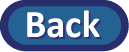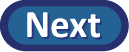## Statistical Inference

Although it is possible that the yawn seed is not more effective (and would have made no difference at all on whether or not someone is going to yawn) and the researchers were lucky and happened to assign more of the subjects who were going to yawn anyway into the yawn seed group, the key question is whether such an outcome is probable

Our null hypothesis in this study is that the yawn seed doesn't make a difference. So as before, we will assume the null hypothesis is true and consider whether the actual observed data is so unlikely to occur "by chance alone" that it provides convincing evidence against the null hypothesis and in favor of the alternative hypothesis (here that the yawn seed increases the probability of a yawn). So we want to hypothetically replicate the study in a world where we know the null hypothesis to be true. That is, we will assume exactly 14 of the 50 people are going to yawn regardless of which group they are put in and any group differences that arise are purely from the randomness introduced by the random assignment process. This will show us what kind of random variation we can expect to see in our statistic when the null hypothesis is true and whether the difference found in the actual study is typical or falls in the tail of the null distribution.

Now the practical question is, how do we do this hypothetical random assignment?  One answer is to use cards:

• Take a set of 50 cards with 14 of one type (e.g., blue) to represent the yawners and 36 of a second type card (e.g., green) to represent those that didn't yawn.
• Shuffle the cards well and randomly deal out 34 to be the yawn seed group (the remaining 16 are the control/no yawn seed group). This replicates the random assignment of the subjects to the "treatment" groups.
• Count how many yawners (blue cards) you have in each group and how many non-yawners (green cards) you have in each group.
• Construct the two-way table to show the number of yawners and non-yawners in each group (where clearly nothing different happened to those in “group A” and those in “group B” – any differences between the two groups that arise are due purely to the random assignment process).

(g) After you are done card shuffling, record the results of your one "could have been" random assignment in the table of your lab report.

(h) Compute the difference in the conditional proportion of yawners in each treatment group for this could-have-been table. (Show your work.)

(i) How does the one "could have been" difference in proportions you simulated in (g) compare to the actual observed difference in conditional proportions found by the Mythbusters (more extreme or less extreme)?